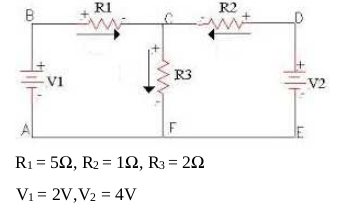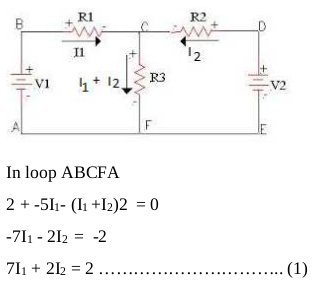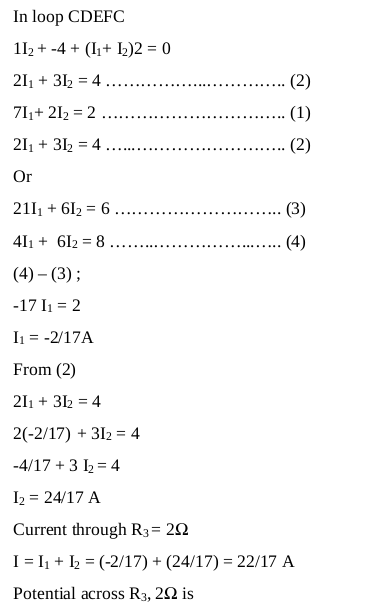# Qn) a) Kirchhoff’s second law is a consequence of               i) Law of conservation of angular momentum               ii) Law of conservation of energy              iii)Law of conservation of charge iv) None of these. b) Find the potential difference across the resistance 2Ω in the given network.a) ii) Law of conservation of energy.

b)V = I R3 =2 × (22/17) = (44/17) V